# Radial distribution function

Jump to: navigation, search

The radial distribution function is a special case of the pair distribution function for an isotropic system. A Fourier transform of the radial distribution function results in the structure factor, which is experimentally measurable. The following plot is of a typical radial distribution function for the monatomic Lennard-Jones liquid.

## Density Expansion of the radial distribution function

The radial distribution function of a compressed gas may be expanded in powers of the density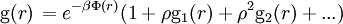$\left. {\rm g}(r) \right. = e^{-\beta \Phi(r)} (1 + \rho {\rm g}_1 (r) + \rho^2 {\rm g}_2 (r) + ...)$

where$\rho$ is the number of molecules per unit volume and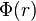$\Phi(r)$ is the intermolecular pair potential. The function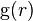${\rm g}(r)$ is normalized to the value 1 for large distances. As is known,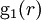${\rm g}_1 (r)$,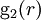${\rm g}_2 (r)$, ... can be expressed by cluster integrals in which the position of of two particles is kept fixed. In classical mechanics, and on the assumption of additivity of intermolecular forces, one has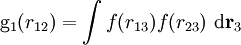${\rm g}_1 (r_{12})= \int f (r_{13}) f(r_{23}) ~{\rm d}{\mathbf r}_3$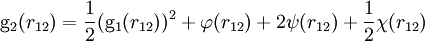${\rm g}_2 (r_{12})= \frac{1}{2}({\rm g}_1 (r_{12}))^2 + \varphi (r_{12}) + 2\psi (r_{12}) + \frac{1}{2} \chi (r_{12})$

where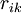$r_{ik}$ is the distance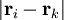$|{\mathbf r}_i -{\mathbf r}_k|$, where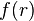$f(r)$ is the Mayer f-function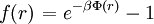$\left. f(r) \right. = e^{-\beta \Phi(r)} -1$

and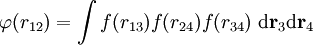$\varphi (r_{12}) = \int f (r_{13}) f (r_{24}) f (r_{34}) ~ {\rm d}{\mathbf r}_3 {\rm d}{\mathbf r}_4$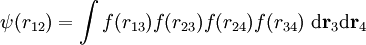$\psi (r_{12}) = \int f (r_{13}) f (r_{23}) f (r_{24}) f (r_{34}) ~ {\rm d}{\mathbf r}_3 {\rm d}{\mathbf r}_4$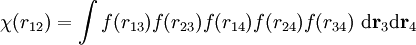$\chi (r_{12}) = \int f (r_{13}) f (r_{23}) f (r_{14}) f (r_{24}) f (r_{34}) ~ {\rm d}{\mathbf r}_3 {\rm d}{\mathbf r}_4$